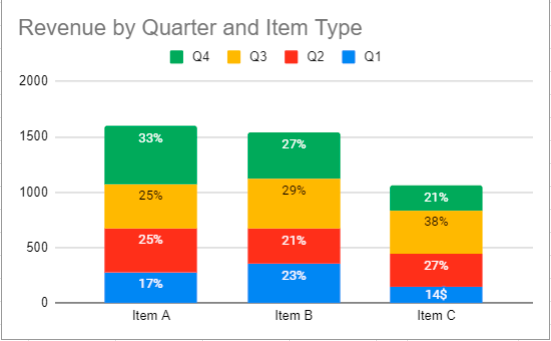# Make a Percentage Graph in Excel or Google Sheets

This graph will demonstrate how to create a percentage graph in Excel and Google Sheets.

## Make a Percentage Graph in Excel

The goal of this tutorial is show how to make a percentage graph based on different datasets.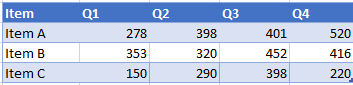### Creating a Stacked Bar Graph

1. Highlight the data
2. Click Insert
3. Select Graphs
4. Click Stacked Bar Graph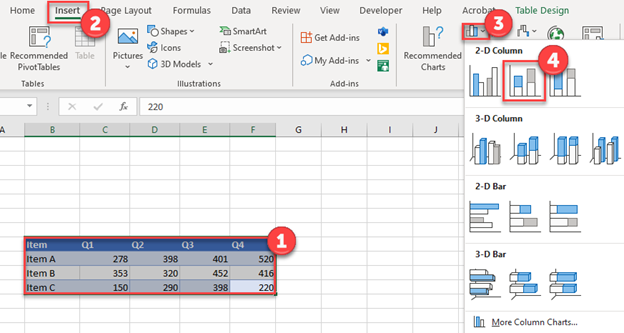Create a SUM Formula for each of the items to understand the total for each.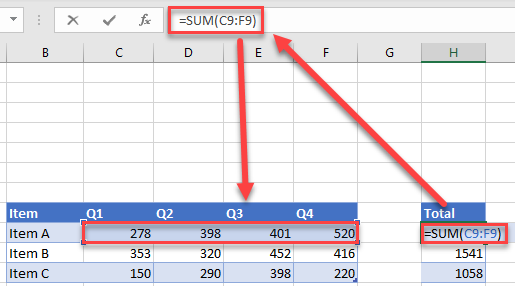### Find Percentages

Duplicate the table and create a percentage of total item for each using the formula below (Note: use \$ to lock the column reference before copying + pasting the formula across the table). Each total percentage per item should equal 100%.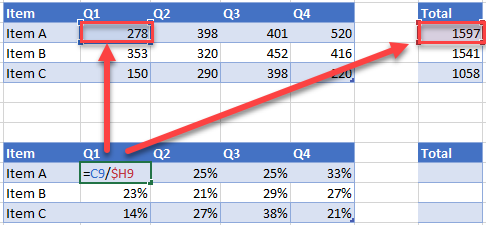### Add Data Labels on Graph

1. Click on Graph
2. Select the + Sign
3. Check Data Labels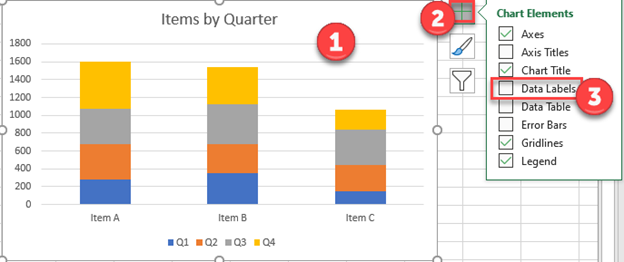### Easy Excel Automation### Change Labels to Percentage

Click on each individual data label and link it to the percentage in the table that was made.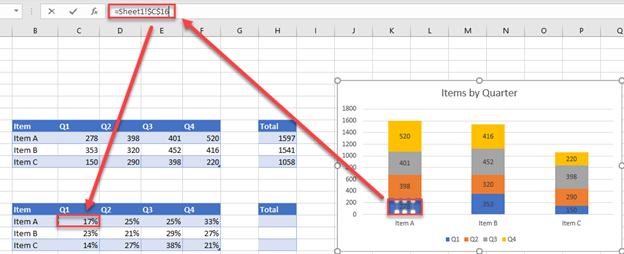### Final Percentage Graph in Excel

The final graph shows how each of the items change percentage by quarter.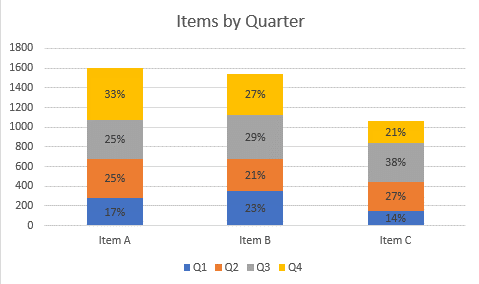## Make a Percentage Graph in Google Sheets

Copy the same data on Google Sheets

### Creating a Graph

1. Highlight table
2. Select Insert
3. Click Chart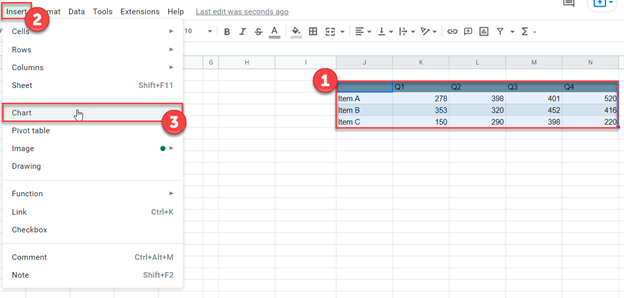If the X and Y Axis need to be swapped, check or uncheck Switch Rows/Columns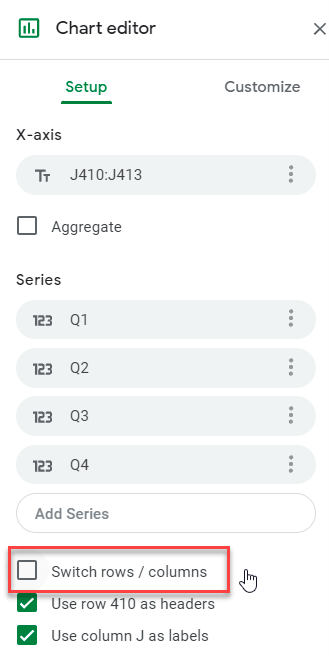### Change Chart Type

1. Select Box under Chart Type
2. Click Stacked Column Chart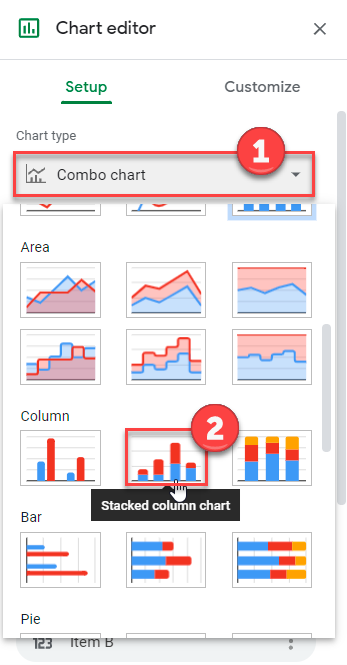1. Click on Customize
2. Select Series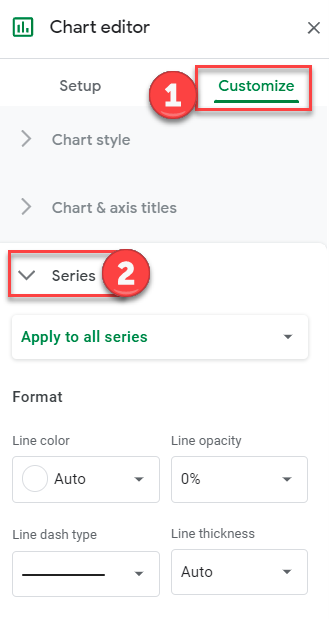3. Check Data Labels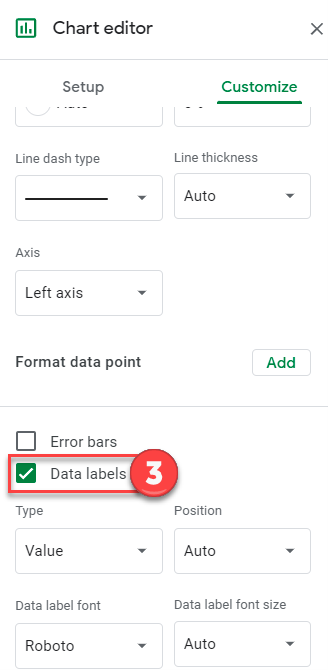Double Click on each Data label and manually edit it to match the percentage (Can’t manually change this based on formula like you can in Excel)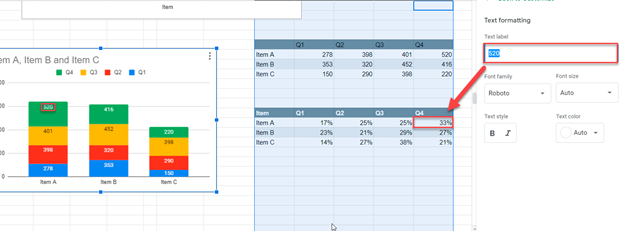### Final Percentage Graph in Google Sheets